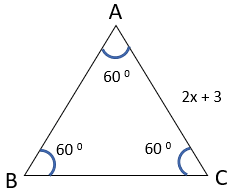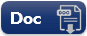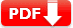# Algebraic Expressions Practice Test Questions Answers

Algebraic Expressions Practice Test Questions Answers: An algebraic expression is formed from variables and constants using different operations. Expressions are made up of terms. A term is the product of factors. Factors may be numerical as well as algebraic (literal). The coefficient is the numerical factor in a term. Sometimes, any factor in a term is called the coefficient of the remaining part of the term.

In general, an expression with one or more than one term (with nonnegative integral exponents of the variables) is called a ‘Polynomial’. To find the value of an expression, we substitute the values of the variables in the expression and then simplify.

Cell Phone Charges For a cell phone plan that charges a monthly fee of $10 plus$0.10 for each minute used, you can use the equation C = 10 + 0.10m to calculate the monthly charges for using m minutes.

## Algebraic Expressions Practice Test

 Test Name Algebraic Expressions Test Prep Type of Question Multiple Choice Question Answers Subject Math Total Question 10 Test Type Sample / Mock Test Editable & Printable PDF / Doc YES (Download link is given below) Available of Answers YES Difficulty Level Elementary Algebra

### Algebraic Expressions Practice Test Question Answers

SET 1

 1. The coefficient of xy in  3x2 zy + 7xyz – 2z2x  is 🔘 A. -5z 🔘 B. 3.5z 🔘 C. -2 🔘 D. 7z 🔘 E. 5yz
 2. The factors of the term –xy2   are 🔘 A. x × y × y 🔘 B. – 1 × y × y 🔘 C. – 1 × x × y 🔘 D. – 1 × x × y × y 🔘 E. NOTA
Answer: D – 1 × x × y × y
 3. Simplify the expression  7x3 – 3x2y + xy2 + x2y – y3 🔘 A.  7x3– 2x2y + xy2 – y3 🔘 B.  7x3– 2x2y + xy2 🔘 C.  7x3– 2x3y 🔘 D.  7x3– 2xy3 🔘 E. 17xy
Answer: A. 7x^3– 2x^2y + xy^2 – y^3
 4. Find the value of the following expressions at a = 1 andb = –2: a3 + a2b + ab2 + b3 🔘 A. 1 🔘 B. 0 🔘 C. 10 🔘 D. -5 🔘 E. -1
Answer: D. -5  = (1)3 + (1)2(–2)+(1) (–2)2 + (–2)3   = 1 – 2 + 4 – 8  = 5 – 10  = – 5
 5. Find each side of an equilateral triangle given below, if it’s perimeter is 240 cm.🔘 A. 38.5 cm 🔘 B. 65.5 🔘 C. 77 cm 🔘 D. 80 cm 🔘 E. 90.5 cm
 6.  Number of terms in the expression 3x2y – 2y2z – z2x + 5 is 🔘 A. -1 🔘 B. 0 🔘 C. 2 🔘 D. 4 🔘 E. -2
 7. Which of the following is a pair of like terms? 🔘 A. –7xy2z, – 7x2yz 🔘 B. – 10xyz2, 3xyz2 🔘 C. 3xyz, 3x2y2z2 🔘 D. 4xyz2, 4x2yz 🔘 E. – 2xyz2, 2x2yz
 Document Type Download Link Free Editable Doc FileFree Printable PDF File